# Electrical Grounding Techniques

An electrical grounding techniques are as follows:
1. Solid grounding
2. Resistance grounding
3. Reactance grounding
4. Arc suppression coil or Peterson coil or resonant grounding
5. Voltage transformer grounding
6. Zig-zag transformer grounding

# Electrical Grounding Techniques

## Solid Grounding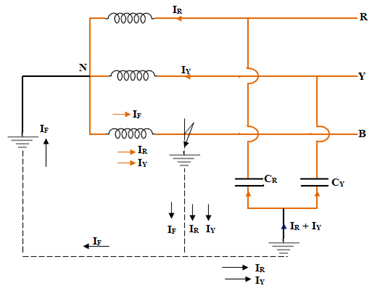In an electrical grounding techniques the solid grounding a direct metallic connection is made as shown on the figure. From the system neutral, one or more earth electrodes consisting of pipes, plates or rod are buried in the ground.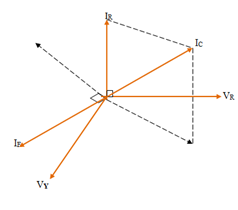1. When an earth fault occurs between earth and any one phase, the voltage to earth of the faulty phase becomes zero. But the healthy phase remains, at their normal phase values. So lightning Arresters of low voltage rating can be used, saving the cost.
2. The flow of heavy fault current IF completely nullifies the effect of the capacitive current IC at the fault and so no arcing ground or over voltages.
3. Due to the flow of high fault current, protective relaying is possible.
4. Increase in earthling fault current causes disturbance in the neighboring communication lines
5. Heavy fault current may damage the circuit breaker contacts.
6. Use of solid grounding is limited to only to systems where the normal circuit impedance is sufficient to prevent very high fault current.

## Resistance Grounding

When it becomes necessary to limit the earth fault current, a current limiting device is introduced in the neutral and the earth path.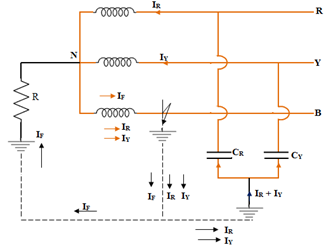One method electrical grounding techniques is to connect a resistance in between the neutral and earth.
• It permits the use if discriminating protective gear.
• Minimizes the hazard of arcing ground
• Due to limitation of fault current, less interference with neighboring communication lines
• Normally used for short lengths of lines where current is small
• Comparatively costlier than solid grounding
• Stability is improved

## Reactance Grounding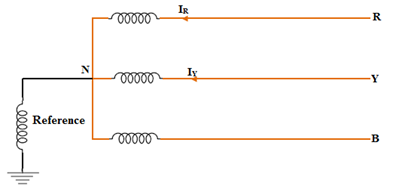Reactance grounding means grounding through impedance, the principal element of which is reactance . For reactance grounding, (Xo\ X1) > 3, but is less than the value for resonant grounding.
Where Xo = Zero sequence reactance
X1 = Positive sequence reactance

For Solid grounding (Xo\ X1) > 3

When a neutral is solidly grounded and if, (Xo\ X1) > 3, then the system is presumed to be reactance grounded. Reactance grounding lies between effective grounding and resonant grounding. The value of reactance keeps the fault current within limits.

This method is used for grounding the synchronous motors and capacitor banks, and for circuits having large charging currents.

## Peterson Coil or Arc Suppression or Ground Fault Neutralizer or Resonant Earthing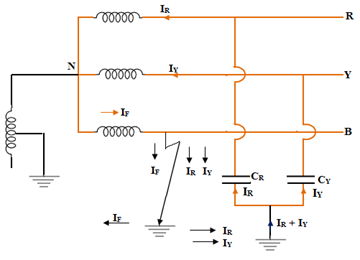An adjustable iron cored reactor (specially constructed) is connected between neutral and earth. Its reactance value is such that the power frequency current between line and earth due to capacitance of healthy lines and earth is equal and opposite to the current in the earth connections. The reactor is used in such resonant earthing is called Peterson coil.

The tapping are used for selection of reactance of Peterson coil depending upon the length of transmission line and hence the capacitance to be neutralized. The reactor is capable of being tuned with the capacitance of the healthy phases to produce resonance when a line to ground (L-G) fault occurs.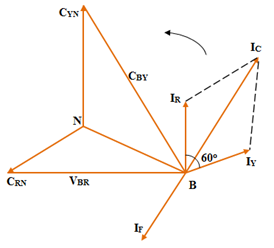It is mainly used to prevent arcing grounds which produce over voltages in the system with ungrounded (isolated) neutral.

The Peterson coil makes arcing ground faults itself extinguishing and in case of sustained ground fault in one of the lines, it reduces the fault current to a very low value, so that the healthy phases can be kept in operation even with one line grounded.

In an under grounded system when a ground fault occurs on any one line, the voltage on the healthy is increases by √3 times (i.e., √3 Vph). Hence the current becomes √3 I per phase, where I is the charging current of line to ground of one phase. The phase sum of the charging currents of the healthy phases becomes 3 times the normal line to neutral charging currents on one phase. Hence,

IC = 3I = { 3Vph / (I / ωC) } = 3Vph ω C

where IC is the charging current.
IL = Vph / ωL

For satisfactory neutralization of arcing grounds, the fault current flowing through the Peterson coil should be equal to IC

IL  = IC.   i.e., Vph / ωL = 3Vph ωC
L = 1 / 3 ω2C
C = Capacitance of line to earth/phase
f = Frequency

For neutral earthing of transformer L.V. Circuit
Zn = V2 / (hxKVAx1000) ohms

where KVA = rating of transformer
Zn = impedance in neutral circuit
L = line Voltage on L.V. side
h = Neutral s/c current in terms of full load line current.

### Arcing Ground

In an ungrounded neutral system under a single line to ground fault, the voltages to earth of the two healthy phases rises from normal phase (Vph) value to line (√3 Vph). The capacitive current in the healthy phases raises to √3 times the normal value and in the faulty phases 3 times the normal value.

A capacitive fault current to earth in excess of about 4A may be sufficient to maintain as arc in the ionize path of the fault, even though the medium which actually caused the fault has cleared off itself. The persistency of the arc due to the flow of capacitive current gives rise to a condition known as “arcing ground” This causes over voltages due to cyclic charging and discharging of system capacitance through the fault currents.

## Earthing Transformer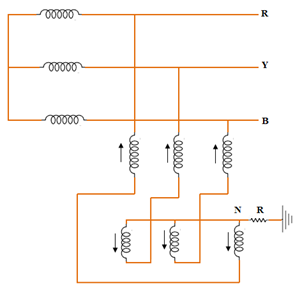If a neutral point is not available or if a neutral point is required a zigzag transformer is used. These transformers do not have secondary winding. Each line of the zig-zag transformer has two identical winding wound differentially such that under normal conditions, the total flux in each limbs is negligible small and so it draws a very little magnetizing current. These transformers are compact in size.

#### Voltage Transformer Electrical Grounding Techniques

If ziz-zag transformer is not available, then voltage transformer is used for the electrical grounding techniques.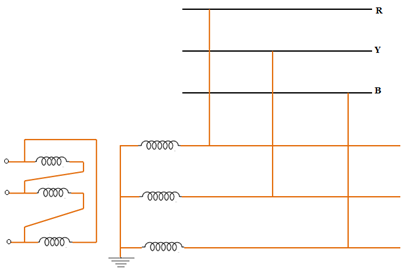0 CommentsComments

## Tags

© 2016 All Rights Reserved